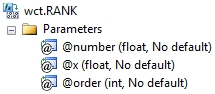# SQL Server RANK function

RANK

Updated: 28 February 2011

Note: This documentation is for the SQL2008 (and later) version of this XLeratorDB function, it is not compatible with SQL Server 2005.

Use the aggregate function RANK to return the rank of a number in a list of numbers. The rank of a number is its size relative to other values in a list.
SyntaxArguments
@number
is the number whose rank you want to find. @number is an expression of type float or of a type that can be implicitly converted to float.
@x
the values to be used in the RANK calculation. @x is an expression of type float or of a type that can be implicitly converted to float.
@order
is a number specifying how to rank @number. If @order is zero or NULL, then RANK is calculated in descending order, Otherwise RANK is calculated in ascending order. @order is an expression of type float or of a type that can be implicitly converted to float.
Return Types
float
Remarks
·         RANK gives duplicate numbers the same rank. However, the presence of duplicate numbers affects the ranks of subsequent numbers. For example, in a list of integers sorted in ascending order, if the number 10 appears twice and has a rank of 5, then 11 would have a rank of 7 (no number would have a rank of 6).
·         If @number is not contained in the dataset, RANK returns an error.
·         @number must remain invariant for the GROUP.
·         @order must remain invariant for the group.
·         RANK is an aggregate function and follows the same conventions as all other aggregate functions in SQL Server.
·         If you have previously used the RANK scalar function, the RANK aggregate has a different syntax. The RANK scalar function is no longer available in XLeratorDB/statistics2008, though you can still use the scalar RANK _q.
Examples

To ascertain where the number 2 ranks, from highest to lowest
SELECT wct.RANK(
2     --@number
,x    --@x
,0    --@order
) as RANK
FROM (VALUES
(1),(2),(2),(2),(2),(3),(4),(5),(6),(7),(8),
(8),(8),(8),(9),(10),(11),(12),(13),(13),(14)
) n(x)

This produces the following result.
RANK
----------------------
17

(1 row(s) affected)

Using the same data, with ranking from lowest to highest.
SELECT wct.RANK(2,x,1) as RANK
FROM (VALUES
(1),(2),(2),(2),(2),(3),(4),(5),(6),(7),(8),
(8),(8),(8),(9),(10),(11),(12),(13),(13),(14)
) n(x)

This produces the following result.

RANK
----------------------
2

(1 row(s) affected)

### SupportCopyright 2008-2021 Westclintech LLC         Privacy Policy        Terms of Service